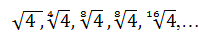Checkout JEE MAINS 2022 Question Paper Analysis : Checkout JEE MAINS 2022 Question Paper Analysis :

# Root 4,....... are the roots of the equation

1) x2 – 4 = 0

2) x2 – 4x + 6 = 0

3) x2 – 5x + 4 = 0

4) x3 – 3x + 2 = 0

Solution: (3) x2 – 5x + 4 = 0

It is given thatare the roots of the derived equation.

x2 – 5x + 4 + 41/2 +¼+⅛+1/16+……. = 0

x2 – 5x + 4 = 0(0)(0)